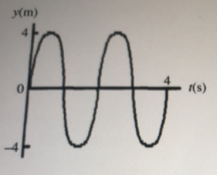# Problem: For the wave shown in the figure, the wavelength isa. 1mb. 4m c. 2md. 8me. unable to be determined

###### FREE Expert Solution

Wavelength:

$\overline{){\mathbf{\lambda }}{\mathbf{=}}\frac{\mathbf{v}}{\mathbf{f}}}$

Frequency, f = 1/T

From the figure provided, the wave makes one complete cycle in 2 seconds.

87% (174 ratings)###### Problem Details

For the wave shown in the figure, the wavelength isa. 1m

b. 4m

c. 2m

d. 8m

e. unable to be determined